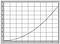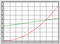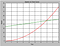Data Visualisation using Matplotlib for beginners.

Making it easy to understand data using insightful and attractive plots!

While solving any data science related problem the first step is to understand the problem statement. Then we acquire data and clean it. Then we do the exploratory analysis for which we use data visualization to get insights. Then comes the modelling and finally we can share the results.

Let’s see how can we visualize data using the following types of plots:

• Line plots
• Scatter Plots
• Bar Graphs
• Pie Charts
• Histograms

Line Plots

To begin let us see how to plot the function y=f(x) on the graph. To do this we need two arrays x and y. where y=f(x). Let us take two functions for y1 and y2.

1.Get the data

x=np.arange(10)
y1= x**2
y2=2*x+34
print(x)
print(y1)
print(y2)
Output---------[0 1 2 3 4 5 6 7 8 9]
[ 0 1 4 9 16 25 36 49 64 81]
[34 36 38 40 42 44 46 48 50 52]

2. Plot Simple curves

To simply plot these functions we use plot function as given below.

plt.plot(x,y1)
plt.show

3. Use themes to make the plot look more attractive

Matplotlib provides some themes to make the plot look more attractive. To get a list of these themes, write the following code.

themes=plt.style.available
print(themes)

Let us try to use one of the theme from this list, say Seaborn-paper. You can try out other themes as well.

plt.style.use(“seaborn-paper”)
plt.plot(x,y1)
plt.show

Output:-Using the seaborn paper theme we got the gray colour border and curve, and grd on the graph.

4. Change the color of curve

Let’s see how to change the color of curve by plotting both y1 and y2 in different colors.

plt.style.use(“seaborn-paper”)
plt.plot(x,y1, color=”red”)
plt.plot(x,y2, color=”green”)
plt.show

Output:-We can plot different curves on same plot using different colors

5. Give titles

Now see how to put titles on your plot.

• plt.xlabel(“ ”): to put a label on x axis.
• plt.ylabel(“ ”): to put a label on y axis.
• plt.title(“ ”): to gve a title to the plot.

See the code and output given below.

plt.xlabel(“Time”)
plt.ylabel(“Speed”)
plt.title(“Speed v/s Time Curve”)We got the label for x and y axis along with the title for curve.

6. Give labels to different curves an plot legend

We use label parameter to gve labels to the different color curves.

plt.style.use(“seaborn-paper”)
plt.plot(x,y1, color=”red”, label=”A”)
plt.plot(x,y2, color=”green”, label=”B”)
plt.legend()
plt.xlabel(“Tme”)
plt.ylabel(“Speed”)
plt.title(“Speed v/s Time Curve”)
plt.show

Output:-

7. Change style of curve

We use linestyle parameter to change the style. Modify this line n the above code as given here.

plt.plot(x,y1, color=”red”, label=”A”, linestyle=”dashed”)

Output:-

8. Putting a marker

To see the points using which we plotted our curve we use marker.

plt.plot(x,y1, color=”red”, label=”A”, linestyle=”dashed”, marker=”o”)

Now you know about some basic parameters used to plot attractive and insightful line plots. Experiment more with different parameters.

“You can achieve simplicity in the design of effective charts, graphs and tables by remembering three fundamental principles: restrain, reduce, emphasize.”